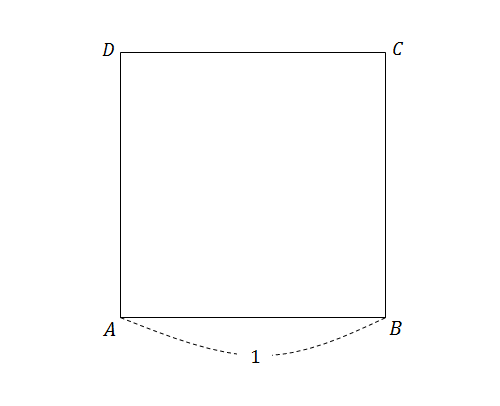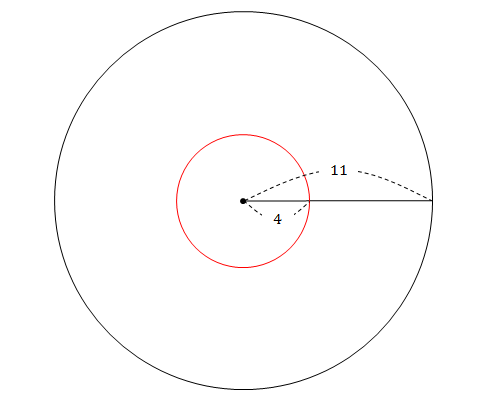Probability

# 2-dimensional Geometric Probability

If we randomly choose two numbers $a$ and $b$ from the interval $[0,1],$ what is the probability that the quadratic $x^2+ax+b=0$ has two distinct real roots?

What is the probability that $x^2+ax+b=0$ has no real roots when $a$ and $b$ are two randomly chosen numbers from the interval $[0,2]?$

Rachel and Ross will go to the gas station at a random time between 4 and 5 o'clock. If it takes exactly ten minutes for each person to refill his/her car and get out of the gas station, what is the probability that they meet at the gas station?In the figure above, $\square ABCD$ is a square with side length 1. Find the probability that $\triangle PAB$ is an obtuse triangle, when $P$ is a randomly chosen point inside $\square ABCD.$The figure above shows the base (of radius 11 cm) of a cylindrical bucket. There is a red circle of radius 4 cm centered at the center of the base. If Tom throws a coin of radius 1 cm into this bucket, what is the probability that the coin will land in contact with the perimeter of the red circle?

×